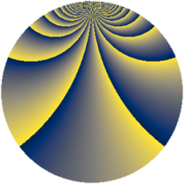# Properties

 Label 1792.2.biLevel $1792$ Weight $2$ Character orbit 1792.bi Rep. character $\chi_{1792}(31,\cdot)$ Character field $\Q(\zeta_{24})$ Dimension $480$ Sturm bound $512$

# Learn more

## Defining parameters

 Level: $$N$$ $$=$$ $$1792 = 2^{8} \cdot 7$$ Weight: $$k$$ $$=$$ $$2$$ Character orbit: $$[\chi]$$ $$=$$ 1792.bi (of order $$24$$ and degree $$8$$) Character conductor: $$\operatorname{cond}(\chi)$$ $$=$$ $$224$$ Character field: $$\Q(\zeta_{24})$$ Sturm bound: $$512$$

## Dimensions

The following table gives the dimensions of various subspaces of $$M_{2}(1792, [\chi])$$.

Total New Old
Modular forms 2176 544 1632
Cusp forms 1920 480 1440
Eisenstein series 256 64 192

## Trace form

 $$480q + 24q^{5} - 8q^{9} + O(q^{10})$$ $$480q + 24q^{5} - 8q^{9} + 16q^{21} - 8q^{25} + 32q^{29} - 48q^{33} + 8q^{37} + 96q^{45} + 40q^{53} - 32q^{57} + 24q^{61} - 16q^{65} - 24q^{73} + 16q^{77} - 48q^{85} - 24q^{89} - 40q^{93} + O(q^{100})$$

## Decomposition of $$S_{2}^{\mathrm{new}}(1792, [\chi])$$ into newform subspaces

The newforms in this space have not yet been added to the LMFDB.

## Decomposition of $$S_{2}^{\mathrm{old}}(1792, [\chi])$$ into lower level spaces

$$S_{2}^{\mathrm{old}}(1792, [\chi]) \cong$$ $$S_{2}^{\mathrm{new}}(224, [\chi])$$$$^{\oplus 4}$$$$\oplus$$$$S_{2}^{\mathrm{new}}(896, [\chi])$$$$^{\oplus 2}$$